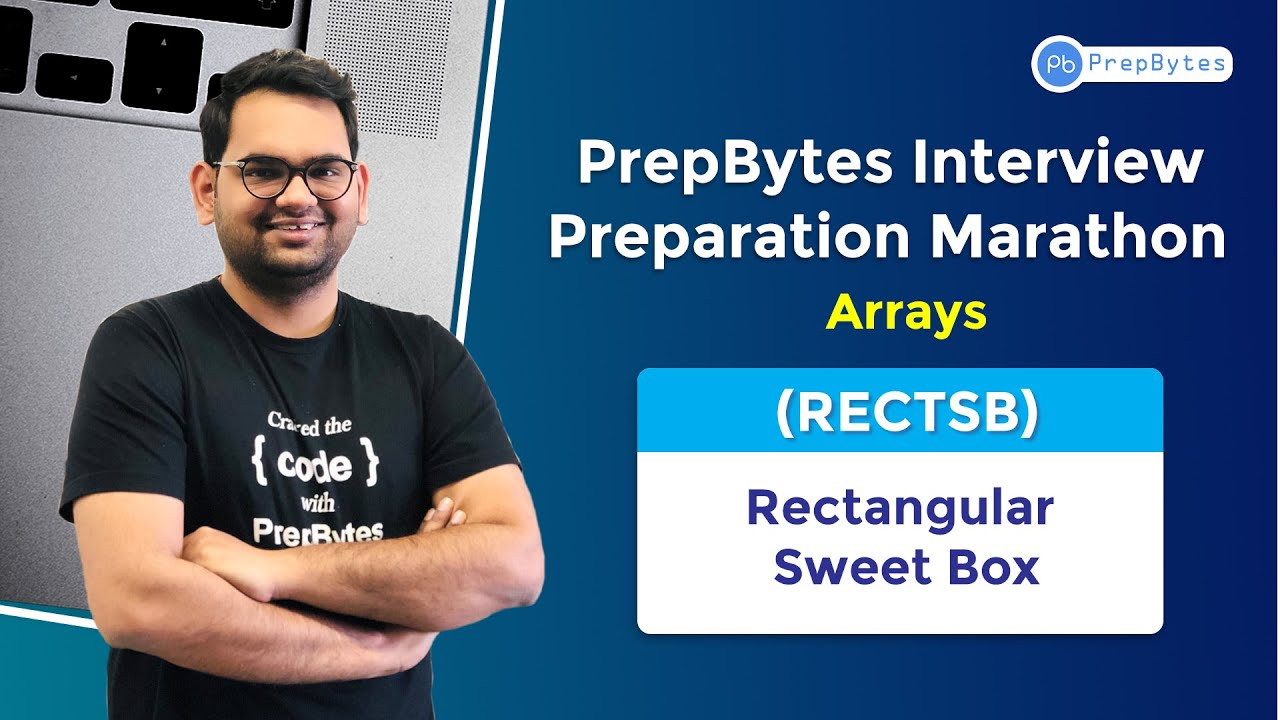Get free ebooK with 50 must do coding Question for Product Based Companies solved
Fill the details & get ebook over emailThank You!
We have sent the Ebook on 50 Must Do Coding Questions for Product Based Companies Solved over your email. All the best!

# Rectangular Sweet Box

Last Updated on March 30, 2022 by Ria PathakPost Min array

Hard

### PROBLEM STATEMENT`(`SIMPLIFIED`)`:

Given an array `A` with `N` elements, your task is to divide the array in `maximum` possible segments such that when these elements are sorted in their individual segments and then all segments are concatenated together, the resultant array should be sorted.

See original problem statement here

Note: Two or more elements can be same.

### For Example:

``````Input : arr = [3 2 4 5 5]

Output : 4

Explanation : As 4 divisions can be made in the array -> [3 2], , , .
If we sort them individually and concatenate in the same order we get [2 3 4 5 5] which is in sorted order.``````

### OBSERVATION :

1. For each division to be valid in the array, the `maximum` value at the left division should be less than or equal to the `minimum` value at the right division. If this condition holds true, we can have such valid divisions.
For Example– Suppose we have `[4 7 6 9 8]`, and we wish to have a partition between `7` and `6`. It is not a valid division as `max` value in the left division i.e. `7` is greater than `min` value in the right division i.e. `6` so after division and concatenation, these divisions will not be sorted properly.

2. So in order to determine whether a division is possible at a particular index say `i`. For each index `i`, we should know the `Maximum` value in the left sub-array and the `Minimum` value in the right sub-array.

### SOLVING APPROACH:

1. The idea is to use `Post Min Array`. This array is used to store the `Minimum` value present at the right of an original array from a particular index.
For Example
`A` = `[5 1 3 2 7 8 6]`
`PostMin` = `[1 1 2 2 6 6 6]`

2. We will construct a `Post Min Array` for our array so that for every index `i`, we know the `Minimum` value that is present at the right of it.

3. For keeping track of `Maximum` value at every index, we will initialize a `Max` value and while traversing the array we will update it for every element.

4. Now we will start traversing the array and checking whether the `Maximum` value at left sub-array is less than or equal to the `Minimum` value at right sub-array. If `Yes` increment `count` by `1`.

### SOLUTIONS:

```#include
#include

int min(int a, int b){
return (ab)? a:b;
}

int maxDivide(int *arr, int n){

/* creating postmin array */
int lmin[n+1];
lmin[n] = INT_MAX;

for(int i=n-1; i>=0; i--){
lmin[i] = min(arr[i], lmin[i+1]);
}

int count = 0;
int v = INT_MIN;

for(int i=0; i
```
```
#include
using namespace std;

int maxDivide(int *arr, int n){

/* creating postmin array */
int lmin[n+1] = {0};
lmin[n] = INT_MAX;

for(int i=n-1; i>=0; i--){
lmin[i] = min(arr[i], lmin[i+1]);
}

int count = 0;
int v = INT_MIN;

for(int i=0; i>t;
while(t--){
int n; cin>>n;
int arr[n];

for(int i=0; i>arr[i];

cout<

```
```
import java.util.*;
import java.io.*;
import java.lang.Math;

public class Main {
static int maxDivide(int []arr, int n){

/* creating postmin array */
int lmin[] = new int[n+1];
lmin[n] = Integer.MAX_VALUE;

for(int i=n-1; i>=0; i--){
lmin[i] = Math.min(arr[i], lmin[i+1]);
}

int count = 0;
int v = Integer.MIN_VALUE;

for(int i=0; i
```
```def maxDivide(arr, n):
lmin = [0 for i in range(n + 1)]
lmin[n] = float("inf")

for i in range(n-1, -1, -1):
lmin[i] = min(lmin[i + 1], arr[i])

count = 0
v = -float("inf")
for i in range(n):
v = max(v, arr[i])
if v <= lmin[i + 1]:
count += 1
return count

for _ in range(int(input())):
n = int(input())
arr = list(map(int,input().split()))
print(maxDivide(arr, n))
```

Space Complexity : `O(N)`, for building `Post Min Array`.

### Refer video for Quick Explanation:[forminator_quiz id="695"]

This article tried to discuss the concept of array. Hope this blog helps you understand and solve the problem. To practice more problems on array you can check out MYCODE | Competitive Programming.